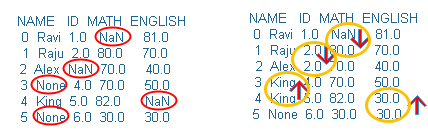# fillna() : Fill NA/NaN values using the specified method

fillna(): Back fill or Front fill NaN or missing data in Pandas DataFrame C#06

We will fill all the NaN and None values with D. Here is the code.
``````import pandas as pd
import numpy as np
my_dict={'NAME':['Ravi','Raju','Alex',None,'King',None],
'ID':[1,2,np.NaN,4,5,6],
'MATH':[np.NaN,80,70,70,82,30],
'ENGLISH':[81,70,40,50,np.NaN,30]}
df = pd.DataFrame(data=my_dict)
print(df) # output without replacing
df=df.fillna('D') # output after replacing
print(df)``````
Output is here , both outputs are given for better comparison.
 print(df) df=df.fillna('D') print(df) `````` NAME ID MATH ENGLISH 0 Ravi 1.0 NaN 81.0 1 Raju 2.0 80.0 70.0 2 Alex NaN 70.0 40.0 3 None 4.0 70.0 50.0 4 King 5.0 82.0 NaN 5 None 6.0 30.0 30.0`````` ``````NAME ID MATH ENGLISH 0 Ravi 1.0 D 81.0 1 Raju 2.0 80.0 70.0 2 Alex D 70.0 40.0 3 D 4.0 70.0 50.0 4 King 5.0 82.0 D 5 D 6.0 30.0 30.0``````
Syntax
``````fillna(self, value=None, method=None, axis=None,
inplace=False, limit=None, downcast=None)``````
Return the Modified DataFrame ( if inplace=True ).
 `value` Value to be replaced. Can be 0. We can use method to replace NaN data also `method` How to use the fill, values are backfill, ffill, pad `axis` 0 or 1 or Column, the axis to be used for replacement `inplace` Boolean , along with method if value is True then original ( source ) dataframe is replaced after applying fillna() `limit` Number , along with method this is the maximum number of replacements allowed. `downcast` what to downcast if possible

## Parameters

We will use options with different valeus to tell how to fill the NaN data.
Each column we will apply different methods to replace the data. Check this code and output below it.
``````import pandas as pd
import numpy as np
my_dict={'NAME':['Ravi','Raju','Alex',None,'King',None],
'ID':[1,2,np.NaN,4,5,6],
'MATH':[np.NaN,80,70,70,82,30],
'ENGLISH':[81,70,40,50,np.NaN,30]}
df = pd.DataFrame(data=my_dict)
print(df)
df['ENGLISH']=df['ENGLISH'].fillna(method='backfill')
df['NAME']=df['NAME'].fillna(method='bfill')
df['ID']=df['ID'].fillna(method='ffill')
print(df)``````For ENGLISH column we have used method='backfill', so the value at row 5 ( value = 30 ) is used at row 4 to replace NaN value.
For NAME column we have used method='bfill' value at 4th row is used to fill value at 3rd row.
For ID column we have used method='ffill' , here value at 1st row is used to fill value at 2nd row.

Note that the last value of NAME column and first value of MATH column is not replaced. ( Why ? )

For MATH column we have used method='pad' , it is same as ffill and as there is no value before it and for NAME column this the last row and there is no data after 5th row ( we used bfill ) , these two data are not changed.

## axis

Using axis=1 we can fill the data in row. We will use method='backfill'
``````my_dict={'NAME':['Ravi','Raju','Alex',None,'King',None],
'ID':[1,2,np.NaN,4,5,6],
'MATH':[np.NaN,80,70,70,82,30],
'ENGLISH':[81,70,40,50,np.NaN,30]}
df = pd.DataFrame(data=my_dict)
df=df.fillna(method='backfill',axis=1)
print(df)``````
Output``````   NAME  ID MATH ENGLISH
0  Ravi   1   81      81
1  Raju   2   80      70
2  Alex  70   70      40
3     4   4   70      50
4  King   5   82     NaN
5     6   6   30      30``````

## limit

Along with method, limit is the maximum number of NaN values are to be replaced. Let us check the code below. As we used axis=0 so in each column only 1 ( limit=1 ) value is replaced.
``````import pandas as pd
import numpy as np
my_dict={'NAME':['Ravi','Raju',None,None,'King',None],
'ID':[1,np.NaN,np.NaN,4,5,6],
'MATH':[np.NaN,80,70,70,82,30],
'ENGLISH':[81,70,40,np.NaN,np.NaN,30]}
df = pd.DataFrame(data=my_dict)
df=df.fillna(method='bfill',axis=0,limit=1)
print(df)``````
Output is here ( note that only one data is replaced in each column ) , the data which are not replaced are highlighted.
``````   NAME   ID  MATH  ENGLISH
0  Ravi  1.0  80.0     81.0
1  Raju  NaN  80.0     70.0
2  None  4.0  70.0     40.0
3  King  4.0  70.0      NaN
4  King  5.0  82.0     30.0
5  None  6.0  30.0     30.0``````

## inplace

By default the value is False. By using inplace=True , the original DataFrame ( source ) is changed. If inplace=False then the original DataFrame is retained.
``````df = pd.DataFrame(data=my_dict)
df.fillna('D',inplace=True)
print(df)``````
Output
``````   NAME ID  MATH ENGLISH
0  Ravi  1    80      81
1  Raju  2    40      70
2  Alex  D    70      40
3     D  4    70      50
4  King  5    82       D
5     D  6    30      30``````

## Counting and identifying NaN values

We can count and display records with NaN by using isnull()

## Removing rows or columns by using dropna()

Rows or columns can be removed by using dropna()

Subscribe to our YouTube Channel here

## Subscribe

* indicates required
Subscribe to plus2netplus2net.com